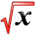# Derivatives of Inverse Trigonometric FunctionsThis article is part of the MathHelp Tutoring Wiki

It is possible to extract the formula required for the calculation of the derivative of inverse trigonometric functions. The following is an example.

Example: Calculate the derivative of function f given by the following,

y = f(x) = arccos(x)

Solution: We are obviously looking for f'(x)=dy/dx. The above equation is rewritten in the following format,

y = arccos(x)

Based on the definition of "arccos" function, it can be concluded that,

x = cos(y);

We differentiate both sides of the above equation with respect to y (it is noted that the derivative of the trigonometric function "cos" is known and is "-sin")

dx / dy = d(cos(y)) / dy and therefore dx/dy = -sin(y)

It can be therefore concluded that dy/dx = -1/sin(y)

we also know that

sin2(y) + cos2(y) = 1 and from the above the cosine can be substituted with x

sin2(y) + x2 = 1 so sin2(y) = 1 - x2 and therefore

dy/dx = -1 / sqrt(1 - x2) = f'(x)